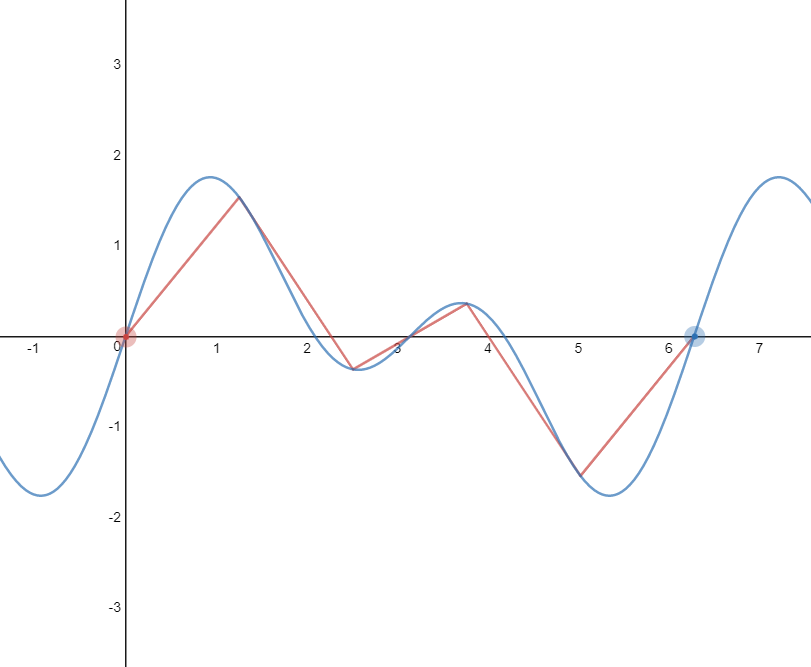# Length of a curve

14 February 2019

After misunderstanding a question in physics, I thought I had to calculate the length of a curved trajectory (ie. the distance) instead of just the straight line (ie. the displacement). Turns out it was the easy option, but I now wanted to know how to get the length of a curve. I mean, it’d just be summing up an infinite number of straight lines, which is almost exactly what integration is…

If you know calculus, you know that there are 2 main concepts:

1. The derivative of a function, which gives you the gradient at a point
2. Integration, which gives you the area under the curve

Now, these are perfectly cool by themselves, but they have some meaning. The gradient is the rate of change, and the area under the graph is the actual change (a quick example with motion). I’m struggling to come up with the meaning of the length of the curve though. But, it’s fun and cool as well…

### The idea

Say you had to do it manually, how would it work? Well, I’d draw lines each at the end of an other on the curve, and then add them all up, and that would give me an approximation. Obviously, the more lines there are, the more precise my approximation would be.Now, we can express this mathematically.

$$\lim_{\delta x \to 0} \sum_{x=a}^b \sqrt{((x + \delta x) - x)^2 + (f(x + \delta x) - f(x))^2}$$

Instead of having a finite amount of lines, I’m just looking at what happens when the interval between each line’s x-distance gets closer and closer to 0 (ie. the lines get smaller and smaller). It’s just Pythagoras basically:

$$d = \sqrt{(x_2 - x_1)^2 + (y_2 - y_1)^2}$$

Now, this is nice, but we can’t do much with this. If you were to compute it, you’d still have to do it manually, and you’d only get an approximation no matter how hard you tried.

But, this does kind of look like an integral do me… I mean there’s a $\lim_{\delta x \to 0}$, a sum, and $\delta x$. Remember:

\begin{aligned} A &= \lim_{\delta x \to 0} \sum_{x=a}^b f(x) \space \delta x \b &= \int_a^b f(x) \dx \end{aligned}

The whole trick is based on remembering first principles for the derivatives:

\begin{aligned} \frac{dy}{dx} &= \lim_{\delta x \to 0} \frac{f(x + \delta x) - f(x)}{\delta x} \b \lim_{\delta x \to 0} \delta x \frac{dy}{dx} &= f(x + \delta x) - f(x) \end{aligned}

Back to our original expression:

\begin{aligned} \text{Length} &= \lim_{\delta x \to 0} \sum_{x=a}^b \sqrt{((x + \delta x) - x)^2 + (f(x + \delta x) - f(x))^2} \b &= \lim_{\delta x \to 0} \sum_{x=a}^b \sqrt{\delta x^2 + \biggr(\delta x \frac{dy}{dx}\biggr)^2} \b &= \lim_{\delta x \to 0} \sum_{x=a}^b \sqrt{1 + \biggr(\frac{dy}{dx}\biggr)^2} \delta x \b &= \int_{a}^b \sqrt{1 + \biggr(\frac{dy}{dx}\biggr)^2} \dx \end{aligned}

Pretty cool hey? For any differentiable $f(x)$, the length of a curve between $a$ and $b$ is given by:

$$L = \int_{a}^b \sqrt{1 + f^\prime (x)^2} \dx$$

Here’s a little desmos to play with the number of lines: